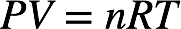# Problem: A sample of gas initially has a volume of 859 mL at 565 K and 2.20 atm.  What is the pressure of the sample if the volume changes to 268 mL while the temperature is increased to 815 K?      A. 9.83 atm      B. 1.05 atm      C. 15.3 atm      D. 6.53 atm      E. 10.2 atm

80% (67 ratings)
###### FREE Expert Solution

Initially, a gas sample has a volume of 859 mL at 565 K and 2.20 atm. We’re being asked to determine the pressure of the gas sample if the volume changes to 268 mL and the temperature changes to 815 K.

Recall that the ideal gas law is:The pressure, volume, and temperature of a gas are related to the number of moles of gas and the universal gas constant:The value nR is constant. For a given moles of gas, the initial and final pressure, volume, and temperature of the gas are related by the combined gas law:80% (67 ratings)###### Problem Details

A sample of gas initially has a volume of 859 mL at 565 K and 2.20 atm.  What is the pressure of the sample if the volume changes to 268 mL while the temperature is increased to 815 K?

A. 9.83 atm

B. 1.05 atm

C. 15.3 atm

D. 6.53 atm

E. 10.2 atm